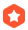# 【＜专题讨论＞［模板模式］＜3＞】SFINAExueweizhong 2003-11-20 10:20:16加精
This "substitution-failure-is-not-an-error" (SFINAE) principle is clearly an important ingredient to make the overloading of function templates practical.

...全文
246 40 打赏 收藏 举报

40 条回复

ajoo 2003-12-31

template<class T>
void f(T t, X<T>* p = 0){
static_assert(false);
}

• 打赏
• 举报

xueweizhong 2003-12-23

• 打赏
• 举报

genvy 2003-12-23

template<class T> void check( sample<sizeof(new T)>* ) { }
template<class T> void check(...) { }

template<class T> void check( sample<1>*,int =sizeof(new T)) { }
template<class T> void check(...) { }

• 打赏
• 举报

genvy 2003-12-23

template <typename T,typename =T::TS> class VValidor;
^^^^^加上这个，让他在substitution时失败

template <typename T,typename> class VValidor
{
public:
typedef int vint;
typedef typename T::TS TS;
};
class Testor
{
public:
typedef int TS;
};
template <typename T> char is_ptr_of_class_defined_TS(T*,typename VValidor<T>::vint =0)
{
//typename T::TS tt;
//UNUSED_VAR(tt);
return 1;
}
int is_ptr_of_class_defined_TS(...)
{
return 0;
}
int main()
{
int i=0;
is_ptr_of_class_defined_TS(&i);
Testor t;
is_ptr_of_class_defined_TS(&t);
return 0;
}
• 打赏
• 举报

xueweizhong 2003-12-23
>to genvy(Leo)

int i=0;
is_ptr_of_class_defined_TS(&i); //int没有定义TS，
// 将会出现编译错误，
// 这刚好是"实例化失败是错误"。
// 这里不发生SFINAE

>to genvy(Paul):

template <typename T>
char is_ptr_of_class_defined_TS(T*,typename T::TS* =0)
^^^^^^^^^^^^^^^^^^^
-----#1
int is_ptr_of_class_defined_TS(...)
-----#2

int i=0;
is_ptr_of_class_defined_TS(&i);

》>to genvy(Paul) && to genvy(Leo) :
1 你的例子没有什么奇怪的地方。

2 另外

template <typename T>
void foo(T); -----------#1
template <typename T>
void foo(T*); ----------#2

3：既然是我没有理解，

• 打赏
• 举报

genvy 2003-12-23

template <typename T> class VValidor
{
public:
typedef int vint;
typedef typename T::TS TS;
};
class Testor
{
public:
typedef int TS;
};
template <typename T> char is_ptr_of_class_defined_TS(T*,typename VValidor<T>::TS =0)
{
//typename T::TS tt;
//UNUSED_VAR(tt);
return 1;
}
int is_ptr_of_class_defined_TS(...)
{
return 0;
}
int main()
{
int i=0;
is_ptr_of_class_defined_TS(&i);
Testor t;
is_ptr_of_class_defined_TS(&t);
return 0;
}
• 打赏
• 举报

genvy 2003-12-23

template <typename T> char is_ptr_of_class_defined_TS(T*,typename T::TS* =0)
{
return 1;
}
int is_ptr_of_class_defined_TS(...)
{
return 0;
}
int main()
{
int i=0;
is_ptr_of_class_defined_TS(&i);
Testor t;
is_ptr_of_class_defined_TS(&t);
return 0;
}
• 打赏
• 举报

genvy 2003-12-23

template <class X> void UNUSED_VAR(X&)
{
}

class Testor
{
public:
typedef int TS;
};
template <typename T> char is_ptr_of_class_defined_TS(T*)
{
typename T::TS tt;
UNUSED_VAR(tt);
return 1;
}

int is_ptr_of_class_defined_TS(...)
{
return 0;
}

int main()
{
int i=0;
is_ptr_of_class_defined_TS(&i); //int没有定义TS，
//应调用is_ptr_of_class_defined_TS(...)
Testor t;
is_ptr_of_class_defined_TS(&t);//定义TS,
//应调用is_ptr_of_class_defined_TS(T*)
return 0;
}
• 打赏
• 举报

ajoo 2003-12-23

• 打赏
• 举报

xueweizhong 2003-12-23

"Daveed的判断是正确的。"

ISO/IEC 14882 : 2003

• 打赏
• 举报

xueweizhong 2003-12-22

template <typename T>
struct error_on_const
{
typedef int type;

typedef error_on_const<T>::const_not_allowed_type
const_not_allowed_type;
//^^^^^^^^^^^^^^^^^^^^^^^^^^^^^^^^^^^^^^^^^^^^^^^^^
//此处是为了导致 “实例化失败就是错误”

};

template <typename T>
struct error_on_const<T const>
//^^^^^^^^^
{
....
};

• 打赏
• 举报

xueweizhong 2003-12-22

auto_ptr的改进，

“SFINAE”（替换失败不是错误）

“实例化失败就是错误”

template <typename T>
struct error_on_const
{
typedef int type;
};

template <typename T>
struct error_on_const
{
typedef int type;

typedef error_on_const<T>::const_not_allowed_type
const_not_allowed_type;
//^^^^^^^^^^^^^^^^^^^^^^^^^^^^^^^^^^^^^^^^^^^^^^^^^
//此处是为了导致 “实例化失败就是错误”

};

template <typename T>
void accept_non_const(T&, error_on_const<T>::type = 0)
{
}

template <typename T>
void accept_non_const(T const&)
{
}

int main()
{
const float foo;
accept_non_const(foo); // error : 因为foo是const 类型
}
• 打赏
• 举报

jhwh 2003-12-22
babysloth(小懒虫虫) ,不懂就别开口。
• 打赏
• 举报

ajoo 2003-12-20

template<class T>void f(T* t){...}

template<class InputIterator, class Predicate>
InputIterator find(InputIterator first, InputIterator last,
Predicate pred,
preicatable<Predicate>::type*=0);

template<InputIterator I, Predicate P>
InputIterator find(I first, I last,
P pred);

> template<class T> void check( sample<sizeof(new T)>* ) { }
> template<class T> void check( int ) { }
>
> int main() {
> check<X>(0);
> return 0;
> }
1。优先解析到check(int)，根本不看check(sample)。没SFINAE什么事。

> template<class T> void check( sample<sizeof(new T)>* ) { }
> template<class T> void check(...) { }

1。优先解析到check(sample)。
2。sizeof(new T)失败，SFINAE生效，这个版本退出竞争，而不是报错。
3。继续解析到check(...)。成功。

• 打赏
• 举报

xueweizhong 2003-12-20
to ajoo:

>你说的“ 因为 sizeof(new T)虽然是无效表达式，
> 但不是无效类型，所以这里不发生SFINAE。”
>是什么意思？既然new T是无效表达式，sizeof(new T)自然也是无效表达式。那么>sample<sizeof(new T)>自然是无效类型了。

• 打赏
• 举报

xueweizhong 2003-12-09

template <typename T>
void find(T); // -------------#1 最一般方式

template <typename Son>
struct Mine : Son {};

template <typename Son>
void find(Mine<Son> const& mine)
{
static_cast<Son const&>(mine).find();
}
//--------------#2 我自己的，
// 为了和#1不产生重载冲突，采用
// CRTP方式

struct Foo : Mine<Foo>
{
void find() {....}
};

• 打赏
• 举报

xueweizhong 2003-11-30

SFINAE不能被安全使用：

1：在函数返回值类型中：

template <typename T>
iterator_traits<T>::value_type
get(T);

int get(int);

int n = get(1);
这个例子在许多编译器上编译出错，而
没有采用SFINAE.

2: 模板类型参数出现在sizeof中。
比如前面帖子中出现过的
template <typename T>
void check(sample<sizeof(new T)>*);

1　的问题更象是编译器的ＢＵＧ，而
2　的问题则更象是c++std的问题，没有说清楚而
导致编译器实现各不相同。
而我的倾向是：
这里的无效值sizeof(new T)
应该能导致sample<sizeof(new T)>是个无效类型，
导致SFINAE失败，导致其永远不能被调用。

SFINAE的情况...期待一下☆☆☆☆☆☆☆★★★★★★

template <typename T>
void copy(T const& source, T& target)
{
...// 一般实现
}

template <typename T>
void copy(T const& source, T& target, POD_has_type<T>::type*=0)
{
memmove(target, source, sizeof(T));
}

T为POD是　POD_has_type<T>::type有定义

• 打赏
• 举报Vue3 中setup()和script setup/script
setup方法中，要将数据暴露给页面模板，需要结合ref,和reactive，并使用return 官网例子： <!-- MyBook.vue --> <template> <div>{{ readersNumber }} {{ book.title }}</div> </...C++类模板 template class T 详细使用方法
C++类模板 template <class T>详细使用方法 类模板与函数模板的定义和使用类似。 有时，有两个或多个类，其功能是相同的，仅仅是数据类型不同，如下面语句声明了一个类： class Compare_int { ...C++类模板 template 详细使用方法
C++类模板 template 详细使用方法 类模板与函数模板的定义和使用类似。 有时，有两个或多个类，其功能是相同的，仅仅是数据类型不同，如下面语句声明了一个类： class Compare_int { public : Compare(int a,...C++类模板template class T简单使用方法vue3 新实验属性 script setup script setup
vue3在单文件组件中引入了一种新的脚本类型示例 <script setup> import { ref } from 'vue' // 像在平常的setup中code // 但是不需要返回任何变量 const count = ref(0)//在此处定义的count可以直接在...

1. 请不要发布与C++技术无关的贴子
2. 请不要发布与技术无关的招聘、广告的帖子
3. 请尽可能的描述清楚你的问题，如果涉及到代码请尽可能的格式化一下